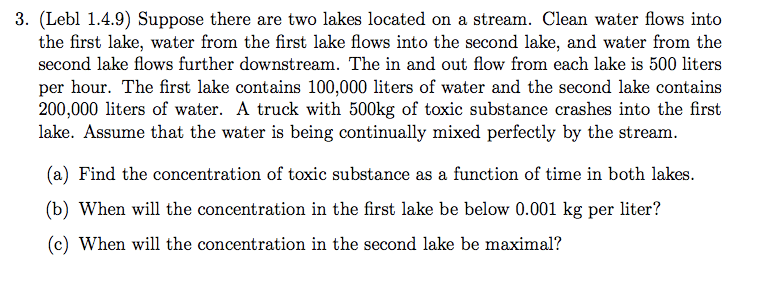# Modelling with differential equations

## Homework Statement## The Attempt at a Solution

I think that problems such as this one tend to take on the rough form of $\frac{dQ}{dt} = rate in - rate out$. I suppose I should treat each lake such that is has it's own equation regarding concentration. I reasoned that, in the case of the first lake, the rate of change in quantity as time changes, call it $\frac{dQ}{dt}$ has no real rate in. In this case, it was more like there was an immediate "lump sum" of toxins released into the lake with no further toxins after that point. Then is the equation for the first lake not given by $\frac{dQ}{dt} = \frac{-Q}{100,000 + 500t}(500)$? I write the +500t because the toxin is becoming more and more diluted as time progresses (because of clean water inflow).

With regard to the second lake's concentration, call it $\frac{dY}{dt}$, it's inflow should be the same as the 1st lake's outflow, no? Thus, write that $\frac{dY}{dt} = \frac{Q}{100,000 + 500t}(500) - \frac{Y}{200,000 + 500t}$.

Now, this is just the way that I tried to reason out how to write the differential equations. Since the rest of the problem relies on these, I think I should get my answers checked before I push onward.

K^2
Your rate-out for the first lake is completely wrong. Lets talk about fixing it, and hopefully, you can figure out the rest.

You want to know specifically how much toxin leaves the lake every hour. You know that 500 liters of fluid flow out. How much of that fluid is water and how much toxin? Lets assume that concentration of toxin in outflow is the same as concentration of toxin in the lake. (So everything is well and properly mixed). The amount of toxin in the lake is Q. The amount of fluid is 100,000 liters. So the concentration is Q/100,000 kg/liter. Now, how much toxin is flowing out? It's the amount of fluid times the concentration. So we get the following.

$$\frac{dQ}{dt}=-\frac{500 Q}{100,000} kg/hr$$

That's it. The fact that concentration drops with time is already accounted for by the fact that Q is decreasing as time goes on, so the value on the right side will be getting lower and lower.

Solve this equation, substitute Q(0)=500kg, and you should know Q(t). Knowing that, you should be able to set up equation for second lake in similar fashion.